Published: 15 February 2016

# Research on sound radiation characteristics of the high-speed train wheel

Li-zong Lin1
Meng-ren Wu2
Zheng-yin Ding3
Hao-wei Gu4
1, 4School of Mechanical and Power Engineering, East China University of Science and Technology, Shanghai 200237, China
2School of Petroleum Engineering, Chongqing University of Science and Technology, Chongqingcity, China
3College of Energy Engineering, Zhejiang University, Hangzhou 310027, China
Corresponding Author:
Meng-ren Wu
Views 89

#### Abstract

Taking the standard wheel model as an example, the radiation noise of a single wheel under excitation force which is computed by multi-body dynamics model is computed by acoustic boundary element method (BEM). Then, the damped wheel is proposed, and the sound radiation characteristics of both wheels are analyzed and compared. The results show that sound field of a single wheel presents an obvious directivity with petaloid change and continuous decrease, and the wheel tread and web contribute the most rolling noise. Compared with the standard wheel, the acoustic radiation power of the damped wheel decreased significantly, especially at the peak frequency. After that, the radiation noise generated by the wheel in the train is researched. The results show that the radiation noise generated by the wheel in the train is a complex sound field after the superposition and interference of multiple wheel noises, which are mainly in the bogies at both ends and its vicinity region. Meanwhile, the basic directivity characteristics of the petaloid change and continuous reduction are remained. The radiation noise which is generated by the wheel in the train has obvious peak characteristic, whose corresponding peak noises are below 110 dB. The radiation noise of the damped wheel is significantly smaller than that of the standard wheel at most frequency bands, and the total SPL at the observation point has decreased by 14.5 dB with obvious noise reduction effect. In order to further research the radiation noise of the damping wheel, influence factors on the noise reduction are analyzed. Finally, these parameters such as thickness and material should be considered comprehensively during designing the damping wheel, in order to find the optimal combination of all parameters.

## 1. Introduction

Wheel-rail noise and aerodynamic noise are two main noise sources in high-speed trains. As shown in the relevant researches, the wheel-rail noise dominates at a certain speed range. Otherwise, the aerodynamic is more significant . The power and exterior shape differences among various trains are greater, and their acoustic conversion speeds are also distinctive. According to the experimental results of French TGV high-speed trains, the wheel-rail noise still dominates when the speed reaches 380 km/h . Therefore, the research on the wheel-rail noise of high-speed trains is very necessary for the treatment and improvement of the noise source.

## 2. Basic theory

### 2.1. Acoustics BEM

According to the acoustical theory, the small amplitude fluctuation of the acoustic wave with the harmonic solution in the homogeneously ideal fluid medium could be expressed as follows:

1
${\nabla }^{2}p\left(x\right)+{k}^{2}p\left(x\right)=0,\mathrm{}\mathrm{}\mathrm{}\mathrm{}x\in D,$

where, ${\nabla }^{2}$ is Laplace operator. $p$ is the sound pressure on the structural surface $x$. $k$ is the number of waves. Sound field $D$ is divided into the external sound field ${D}^{+}$ and internal sound field ${D}^{-}$, and their boundaries are $\partial {D}^{+}$ and $\partial {D}^{-}$, respectively.

The wheel radiation noise can be computed by means of the direct BEM . Namely, the above differential equation is solved under the given boundary conditions. Finally, the sound pressure at any point $x$ in the external sound radiation field as well as the relationship between the sound pressure and sound pressure gradient value is obtained as follows:

2
$p\left(x\right)=\underset{s}{\iint }\left[p\left(y\right)\frac{\partial G\left(x,y\right)}{\partial \mathbf{n}}-G\left(x,y\right)\frac{\partial p\left(y\right)}{\partial \mathbf{n}}\right]dS\left(y\right),$

where $p\left(x\right)$ and $p\left(y\right)$ represent the sound pressure at points $x$ and $y$, respectively. $\mathbf{n}$ is the normal vector on the surface $S$. $G\left(x,y\right)$ is Green function of the free space.

### 2.2. Rigid multi-body dynamics

During operation of a high-speed train, the wheel-rail force between wheels and the rail is produced due to the irregularity between them. On one hand, the force excites the vibration between wheels and the rail directly, thus generating wheel-rail noise. On the other hand, the force is transferred to the train body to cause structural vibration response. Under the irregularity excitation of the rail, the dynamic equation of the train is as follows:

3
$\mathbf{M}\stackrel{¨}{\mathbf{y}}+\left({\mathbf{C}}_{s}+{\mathbf{C}}_{r}\right)\stackrel{˙}{\mathbf{y}}+\left({\mathbf{K}}_{s}+{\mathbf{K}}_{r}\right)\mathbf{y}=\mathbf{B}\mathbf{u},$

where $\mathbf{M}$ is the inertia matrix. ${\mathbf{C}}_{s}$ and ${\mathbf{C}}_{r}$ are damping matrixes caused by the suspension system and wheel-rail, respectively. ${\mathbf{K}}_{s}$ and ${\mathbf{K}}_{r}$ are the stiffness matrixes caused by the suspension system and wheel-rail, respectively. $\mathbf{B}$ is the distributed matrix input by the rail, and $\mathbf{u}$ is the input vectors of the irregularity.

When the high-speed train is driving at a constant speed straightly, the wheel-rail force of the train is primarily vertical, which can be predicted based on the train-rail coupling and rigid multi-body dynamics . Its related computation formula is as follows:

4
$F\left(\omega \right)=-\frac{R\left(\omega \right)}{{\alpha }^{W}\left(\omega \right)+{\alpha }^{C}\left(\omega \right)+{\alpha }^{R}\left(\omega \right)},$

where, $F$ is the vertical wheel-rail force. $\omega$ is the circular frequency. $R$ is the roughness. And ${\alpha }^{W}$, ${\alpha }^{C}$ and ${\alpha }^{R}$ are the displacement admittances (ratio of the force to displacement) of the wheel, contact spring and the rail, respectively.

## 3. Computation of wheel radiation noise

### 3.1. Multi-body dynamics model

Before computing wheel radiation noise, the wheel-rail force which is applied on the wheels need to be obtained.

High-speed train head is a complex multi-body vibration system, mainly including the body, bogie, wheel sets, and axle box, etc. Therefore, some elements need to be simplified during building the train model, in order to simulate the excitation force produced by the train on the irregular rail.

Table 1Rigid-body parameters of the coach

 Physical parameters Values Physical parameters Values Body mass 38.9 t One-line longitudinal rigidity 920 kN/m Rolling inertia 126 t·m2 One-line transverse rigidity 920 kN/m Nodding inertia 1905 t·m2 One-line vertical rigidity 886±60 kN/m Shaking inertia 1798 t·m2 Two-line transverse rigidity 125 kN/m Height of gravity center 1.66 m Two -line vertical rigidity 195 kN/m

By means of the professional multi-body dynamics software SIMPACK, the rigid models of the bogie, wheel sets, axle box and body are established through the sub-structure and parametric modeling approaches. In addition, the corresponding mass and moment of inertia are given as Table 1. The junction is adopted to simulate the connection type of each component. The force element is used to simulate the flexible elements of springs and dampers, and the rigidity and damping are given. The vehicle-rail coupling dynamics model is shown in Fig. 1.

Fig. 1The vehicle-rail coupling dynamics model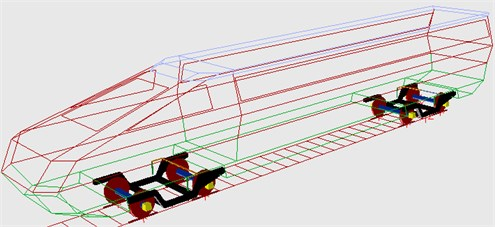The train is at the speed of 350 km/h, and the rail has applied U.S. 5-level route spectrum. It is defined that the bogie near the head is bogie 1, and the one near the tail is called bogie 2. Through the multi-body dynamics simulation, the excitation force which will be applied on the wheel of bogie 1 is as Fig. 2. In addition, the excitation force applied on wheels of bogie 2 is similar to that of bogie 1.

As seen from Fig. 2, the longitudinal excitation force on the wheels is far less than the other directions. In addition, the excitation forces on four wheels differ little, which is consistent with the actual situation because both high-speed trains and wheel rails are symmetrical structures.

Fig. 2Excitation force of contact points at speed of 350 km/h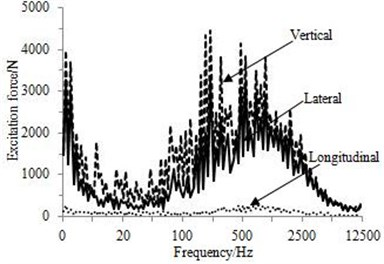a) A point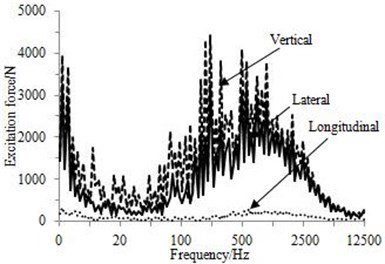b) B point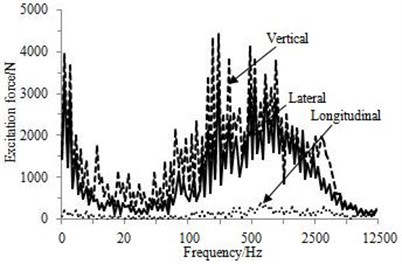c) C point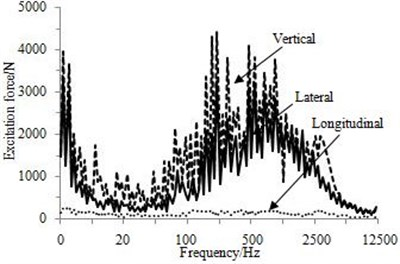d) D point

### 3.2. Standard wheel model

The sectional properties of the wheel researched in the paper are shown as Fig. 3(a). Its radius is 465 mm and the applied web is straight with the thickness of about 35 mm. To ensure the accuracy of the simulation, the finite element is primarily divided to hexahedron element, as shown in Fig. 3(b). The number of elements of a single wheel is about 26,000. In terms of wheels, the material is steel, and the elastic modulus, density and Poisson’s ratio are 2.1×1011 Pa, 7800 kg/m3 and 0.3, respectively. As the damping of steel is very small, its influence on the wheel vibration and radiation noise is not yet considered.

In order to learn about the sound radiation properties of a single wheel preliminary, the boundary conditions are made the following simplification in the paper. 1) The finite element model of the axle is not established and only the fixed constraint is defined in the hub hole so as to simulate the connection between the axle and wheels. 2) During operation, the longitudinal excitation force applied wheels of the train is negligible. The contact point between the wheel tread and rail is the action point.

Fig. 3Sectional propertied and finite element model of wheels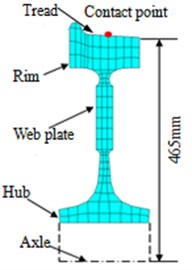a) Sectional properties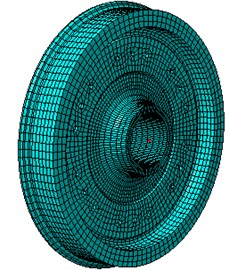b) Finite element model

Table 2Comparison of the experimental modal and simulation modal

 Order Simulation value / Hz experimental value / Hz Relative error / % 1 134.7 138.2 –2.51 2 192.8 190.5 1.21 3 356.1 354.7 0.39 4 533.4 536.2 –0.52 5 956.6 963.1 –0.67 6 1287.8 1280.4 0.57

Based on the above finite element model, the top 6-order modals are computed under constrain condition as Fig. 4. It can be seen that the modal shapes at every order are symmetrical.

As the finite element model of the wheel is relatively complex, it is necessary to conduct experiment and verify the accuracy of the model in order to ensure the reliability of the subsequent analysis.

56 measuring points are arranged at the web plate and tread of the wheel, and the force hammer is used radially and axially on the wheel tread and rim, respectively, as shown in Fig. 5. The acceleration sensors are used to test the responses of 56 points, wherein the measuring points on the wheel web plate are aimed at testing their responses to axial acceleration while the points on the wheel tread are aimed at that of the radial direction. Finally, Test.lab is applied for data collection and processing, with the sampling frequency of 1.28 kHz. Each measuring point is hammered three times in the testing, and the hammering signals and response signals to the acceleration obtained each time are taken the average value. At last, the experimental result of wheels can be obtained and compared with the simulation value, as Table 2.

As can be seen from Table 2, the relative error between the experimental and simulation modal is controlled within 5 %, which indicates that the finite element model is reliable.

Fig. 4Top 6-order constraint modal shapes of the wheels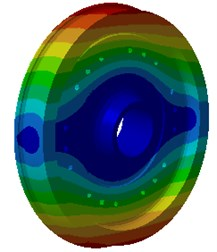a) 134.7 Hz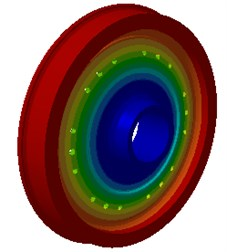b) 192.8 Hz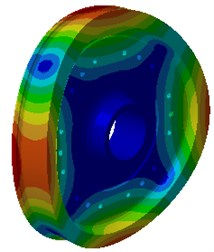c) 356.1 Hz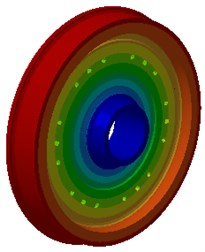d) 533.4 Hz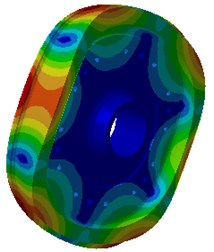e) 956.6Hz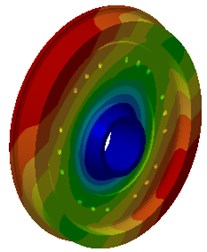f) 1287.8 Hz

Fig. 5Hammering diagram of the wheel modal test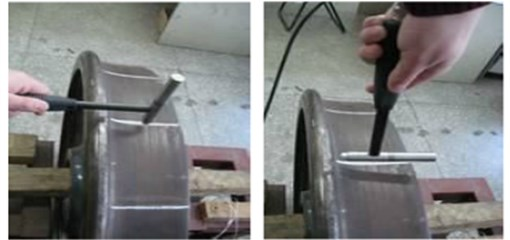### 3.3. Radiation noise of the standard wheel

Under the effect of the wheel-rail excitation force, the generated vibration response of the standard wheel is set as a boundary condition. Based on acoustic BEM, the radiation noise of wheel can be computed. In the paper, the simulation frequency band is 0-3000 Hz and the step length is 10 Hz. The surface of the finite element mesh model is extracted to establish the boundary element mesh model as Fig. 6, whose size is in accordance with the 1/6 wavelength principle. In order to prevent sound leakage from the hub hole, an additional element is adopted to seal the hole. Meanwhile, three plane field points are built based on the reference coordinate system in order to observe the radiated sound field of wheels, as Fig. 7.

Fig. 6Boundary element mesh model of wheels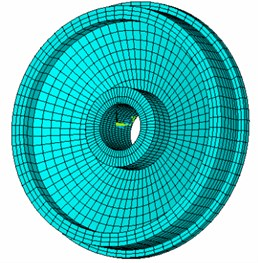Fig. 7Plane field point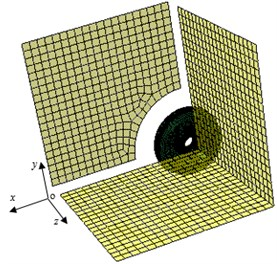Fig. 8Sound power spectrum of the standard wheel under wheel-rail excitation force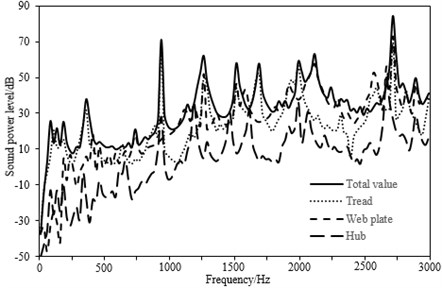The modal shape and radiation sound field contour of the standard wheel at 960 Hz, 1670 Hz, 2710 Hz and 2890 Hz are shown in Fig. 9. The original point $o$ of coordinates is the wheel center, the plane $oxy$ is parallel to the wheel rolling direction, the plane $oyz$ is the vertical surface, and the plane $oxz$ is its horizontal plane, as shown in Fig. 7. As modals at 960 Hz and 1670 Hz are mainly presented as the bending vibration of the rim, the radiation noise distribution on the vertical plane $oyz$ is relatively large. As modals at 2710 Hz and 2890 Hz are mainly presented as the radial stretch of the tread, the noise is seriously distributed in planes $oxy$ and $oyz$.

### 3.4. Radiation noise of the damped wheel

The wheel radiation noise can be reduced by improving the main noise source. As known from Fig. 8, the tread and web plate contribute the most sound radiation power. Due to its relationship with the matching of wheels and rail profile and the impacts on the operation safety, the improvement workload regarding tread is larger and its feasibility is still unclear. The low-noise control can be considered for the web plate, and applying damping is the most commonly method to reduce noise .

Fig. 9Modal shape and radiation sound field of the standard wheel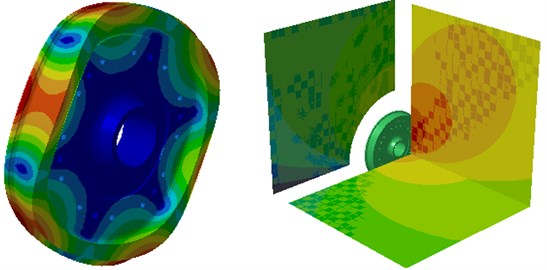a) 960 Hz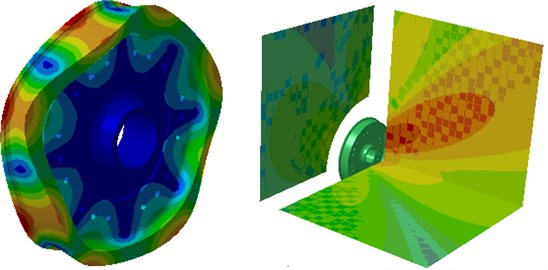b) 1670 Hz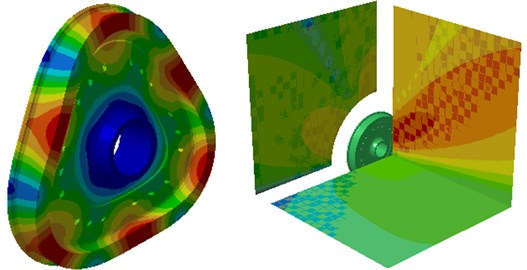c) 2710 Hz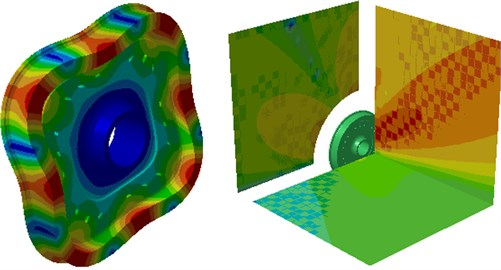d) 2890 Hz

The geometric structure of the damping wheel is shown as Fig. 10(a), and it is consisted of a fan-like damping structure with 6 equal portions. Each structure is composed of 4 layers steel plates and 3 layers damping plates, with the plate thickness of about 2 mm. The plate and the wheel are connected and fixed by the mounting rings, including big and small rings located in the rim and the exterior hub of the basis wheel, respectively. The corresponding finite element model is shown in Fig. 10(b), the connections between the plate and plate, the plate and the mounting ring, as well as the mounting ring and basis wheel are all simplified into the common node relation. Steel is chosen as the material of the basis wheel, ZN03 damping material is selected for the damping plate, and the relevant material parameters can be found in Table 3.

Fig. 10Geometric structure and finite element model of the damped wheel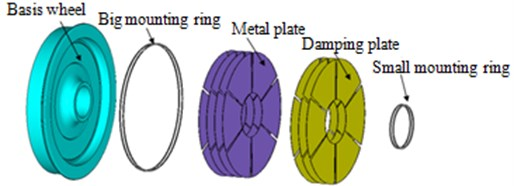a) Geometric structure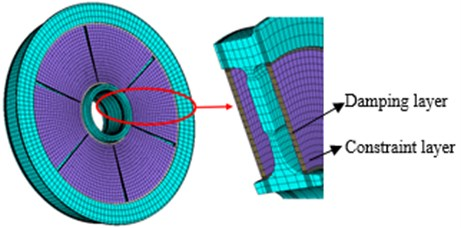b) Finite element model

Table 3Material parameters of the damped wheel

 Different material Elastic modulus N/m2 Density Kg/m3 Poisson’s ratio Mean value of damping loss factor Steel 2.10×1011 7800 0.3 0.001 ZN03 1.5e7 1000 0.4 0.5

Fig. 11Comparison of sound radiation power between the standard wheel and damped wheel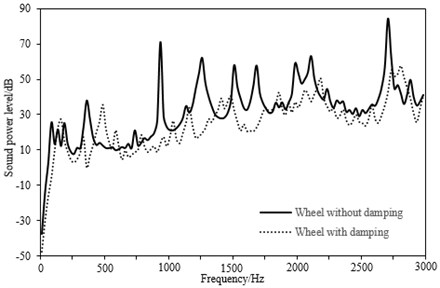Similarly, acoustic BEM is applied to compute the sound radiation power of the damped wheel and it was compared with that of the standard wheel, as shown in Fig. 11. Firstly, in terms of frequency, the overall stiffness of the wheel is increased and the modal frequency is raised after applying damping plate. Due to the larger radiation noise at modal frequencies, the peak frequency regarding the sound radiation power of the damped wheel is moving to higher frequency when it is compared with that of the standard wheel. Meanwhile, the increase of local modals of wheels will also be caused by damping plate, thus leading to more peak frequency on the sound radiation power frequency spectrum of the damped wheel. Secondly, with respect to the amplitude, the sound radiation power of the damped wheel is nearly improved throughout the analysis band when it is compared with that of the standard wheel. Among them, the noise peaks at the overall modal frequencies of each order are significantly decreased, while noise peaks caused by the local modals are relatively small and their contribution to the total sound radiation power also can be ignored. In general, better noise reduction effect is presented from the damped wheel.

## 4. Radiation noise in the high-speed train

The sound radiation characteristics of single wheel are researched and the damped wheel is then proposed, which have improved the radiation noise to a certain extent. Further researches are required to understand the influence of this improvement on the high-speed train.

### 4.1. Radiation noise computation in the high-speed train

Currently, the computational methods of the interior noise include the finite element and boundary element method. Due to the larger geometric size and more meshes of the high-speed train, the computation efficiency of the finite element method is relatively low. Therefore, BEM is chosen in this paper to compute the noise in the high-speed train.

As shown in Fig. 12, BEM of the high-speed train is built with a total of 23,851 elements and 32,169 nodes.

Fig. 12BEM model of the high-speed train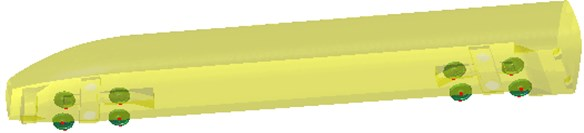Fig. 13Comparison of radiation noise in the train surface at 1100 Hz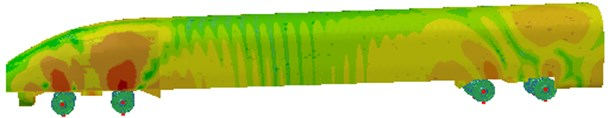a) Radiation noise of the standard wheel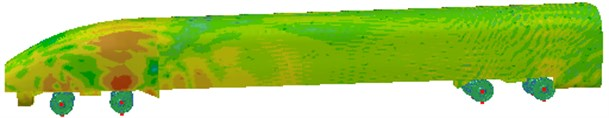b) Radiation noise of the damped wheel

The standard wheel radiation noise on the train body surface at 1100 Hz is shown in Fig. 13(a). It can be seen that the radiation noise of the wheel in the middle position is relatively small, and the positions with larger acoustic response are bogies and its vicinity region. The radiation noise of four wheel sets can be seemed as a complex sound field after the superposition and interference of multiple wheel noises, and the acoustic responses may be enhanced or weakened at different positions. However, the characteristics of petaloid distribution and reduction layer-by-layer are presented at ends of the train, similar to the free radiation sound field of a single wheel. The same method is used to compute the radiation noise of the damped wheel, whose sound field contour at 1100 Hz is shown as Fig. 13(b). Seen from the comparison result, the noise reduction of the damped wheel is very significant. Moreover, the distribution characteristics are still remained, which indicates that the noise directivity cannot be changed by applying damping in the standard wheel.

Specifically, radiation noise of an observation point at the ends of the train is extracted, as shown in Fig. 14. It is shown that the wheel radiation noise is mainly focused on the medium and high frequencies above 1000 Hz, with obvious peak characteristics. Peak noises are all below 110 dB, but the size relationship between peaks is different from the sound radiation power of the standard wheels in Fig. 8, which is mainly due to the obvious directivity of the radiation sound field for a single wheel. After the superposition and interference of many wheel noise sources, the noise response at different frequencies will be strengthened or weakened, thereby changing the original amplitude features. By comparison, it could be seen that the radiation noise of the damped wheel moves towards the higher frequency in terms of the peak frequency due to the influence of wheel stiffness, but the noise amplitude has decreased almost in the entire frequency band. With respect to the total value, the radiation noises of the standard wheel and damped wheel at the observation point are 111.2 dB and 96.7 dB, respectively, with a difference of 14.5 dB, indicating that there is a significant improvement of the damped wheel on the radiation noise.

Fig. 14SPL comparison at the observation points on the train surface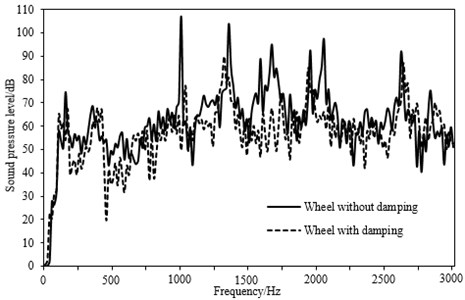### 4.2. Analysis for influence factors on the radiation noise of damping wheels

As can be seen from the above analysis, the radiation noise on the train surface can be effectively reduced by laying the damping structure on wheels, and the corresponding type of the damping wheel is shown in Fig. 10. However, although the radiation noise is decreased compared with the previous result, the absolute value is still large. Therefore, it is necessary to research influence factors on the radiation noise of damping wheels, in order to design an optimal structure.

There are many factors affecting the noise reduction of the damping wheel. In the reference , different positions for the damping layer are considered to research their effects on the noise reduction of wheels. Besides positions, the materials and thicknesses of damping layers and constraint layers will also have a greater effect on the noise reduction effect of damping wheels. Damping wheels with 6 cases are researched in this paper, and the parameters of each wheel is displayed in Table 4. By means of the above analysis method, the radiation noise of damping wheels on the train surface is computed under each case.

Cases 1, 2 and 3 are chosen to compare with the effects of damping layer thickness on the noise reduction of the damping wheel, cases 2, 4 and 6 are chosen to compare with the constraint layer thickness on the noise reduction of the damping wheel, and cases 4 and 5 are chosen to compare with the effects of constraint layer material on the noise reduction of the damping wheel. The radiation noise on the train surface under different cases are then computed and compared, which are shown in Fig. 15(a), (b) and (c), respectively.

Fig. 15Radiation noise of the damping wheel under different structural parameters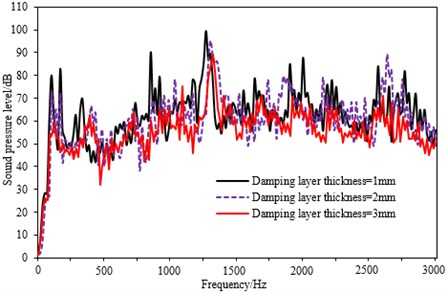a) Cases 1, 2 and 3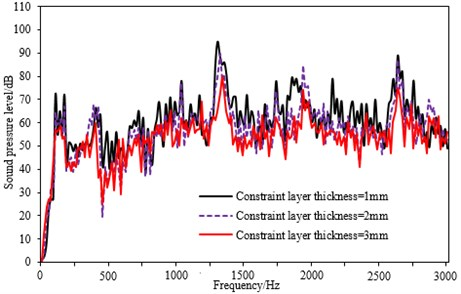b) Cases 2, 4 and 6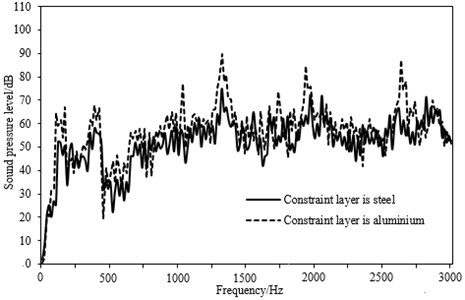c) Cases 4 and 5

As can be seen from Fig. 15(a), the thicker the damping layer is, the smaller the radiation noise of the damping wheel will be in many frequencies. It can be explained by that the attenuation effect of the damping layer will be improved with the increasing of the damping layer thickness, and then the vibration generated from the wheel will be dissipated to a great degree through the damping layer. It is seen from Fig. 15(b) that the effect of the changing constraint layer thickness is similar to that of the damping layer, namely, with the increase of the thickness, the radiation noise of the wheel will be reduced. That is because the greater the constraint layer thickness is, the overall stiffness of the wheel will be increased, and the damping dissipation energy generated in the vibration process of the wheel will be also higher due to the shear deformation. As known from Fig. 15(c), the radiation noise of the damping wheel will be reduced when the constraint layer material is replaced by steel with equal thickness. That is mainly because the elasticity modulus of steel is greater than that of aluminum under the same thickness. As a result, the rigidity of the entire wheel will be larger, and the damping dissipation energy in the vibration process of the wheel will be also higher due to the shear deformation. Therefore, these parameters should be considered comprehensively during designing the damping wheel, in order to find the optimal combination of all parameters.

Table 4Parameter of damping wheels under each case

 Cases Damping layer Constraint layer Material Thickness Material Thickness Case 1 ZN03 1 mm Aluminum plate 1 mm Case 2 ZN03 2 mm Aluminum plate 1 mm Case 3 ZN03 3 mm Aluminum plate 1 mm Case 4 ZN03 2 mm Aluminum plate 2 mm Case 5 ZN03 2 mm Steel plate 2 mm Case 6 ZN03 2 mm Aluminum plate 3 mm

## 5. Conclusions

1) Modal experiment is conducted for the standard wheel, which are compared with the computational modals of the finite element. The relative error is controlled within 5 %, indicating that the finite element model is reliable and can be used for the subsequent analysis.

2) The radiation noise of the standard wheel is mainly concentrated in the medium and high frequency above 1000 Hz. The wheel treads and web plate contribute the most noise. An obvious directivity is shown in the radiation sound field with petaloid change and continuous decrease.

3) Not only part radiation noise generated by the web plate could be shielded directly by the damped wheel and dissipate the vibration energy as heat, but also the radial movement of the entire wheel can be inhibited. Therefore, compared with the standard wheel, its sound radiation power is significantly lower within 3000 Hz.

4) The radiation noise which is generated by the wheel in the train surface is a complex sound field after the superposition and interference of multiple wheel noises, which are mainly in the bogies at both ends and its vicinity region. Meanwhile, the basic directivity characteristics of the petaloid change and continuous reduction are remained.

5) The radiation noise which is generated by the wheel in the train surface has obvious peak characteristic, whose corresponding peak noises are below 110 dB. The radiation noise of the damped wheel is significantly smaller than that of the standard wheel at most frequency bands, and the total SPL at the observation point has decreased by 14.5 dB with obvious noise reduction effect.

6) In order to further research the radiation noise of the damping wheel, influence factors on the noise reduction are analyzed. Finally, these parameters such as thickness and material should be considered comprehensively during designing the damping wheel, in order to find the optimal combination of all parameters.